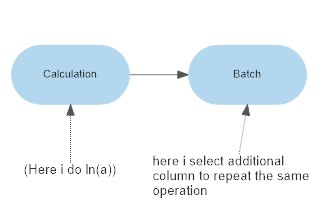Repeated calculation

Hello
assuming that I have 100 columns of data. I need to log each of them. Is there a simple way to do it?
Thanks

I’m not sure what you mean. Can you post a simplified example of the input you have and the corresponding output you want.

This is a hypothetical question. Here I have a really simple example:

|a |b |c|d|
|1|2|3|4|
|2|3|4|5|
|3|4|5|6|
|4|5|6|7|
|5|6|7|8|
|6|7|8|9|

I have four Columns: a, b, c d. I want to have four more columns: ln(a), ln(b), ln(c) and ln(d). In other words, I want to do ln for each column.
Essentially, this problem is related to the calculation function. It would be better for it to have a selection panel like in the “remove cols”. We select multiple columns, and do the same operation for each column.

Easy, peasy like most things in EDT. Lookup the Javascript transform. I believe your use case is an example.

You would have to do this as 4 separate transforms using either Calculate or Javascript:

I can see that would be useful for unary operations like ln (that operate on 1 column). But some calculations require 2 columns as input (e.g. Multiply), which complicates things. I will give it some thought

A more powerful way is to allow some kind of programming. For example, something like

for column “a” to “d”:
{add new col = ln(current column)}
the problem is, of course, which programming language to use, and how to incorporate the function of EDT into it.

This might be possible in future with a more powerful form of the Javascript transform. Perhaps for v2.

Another thought: can we have a “batch for function” function.
For example, I perform a calculation function. After the calculation function, we have a batch function. In the batch function, we can select column or row that we want to repeat the previous function. it will looks like thisthe advantage here is that it will allow more complicated, Excel style operation. For example, you can have two column add together. In the batch function, the user can select multiple first columns for the add operation. The program can do the calculations like you copy and paste it in Excel.

I’m not sure that fits in very well with the way Easy Data Transform is designed. But I understand the requirement to perform the same calculation on lots of columns and I will give it some thought.

Thinking about it a bit more, it could allow selecting multiple columns for unary operations and a pair or columns (or a column and a constant value) for binary operations. It is a posibility for future releases.